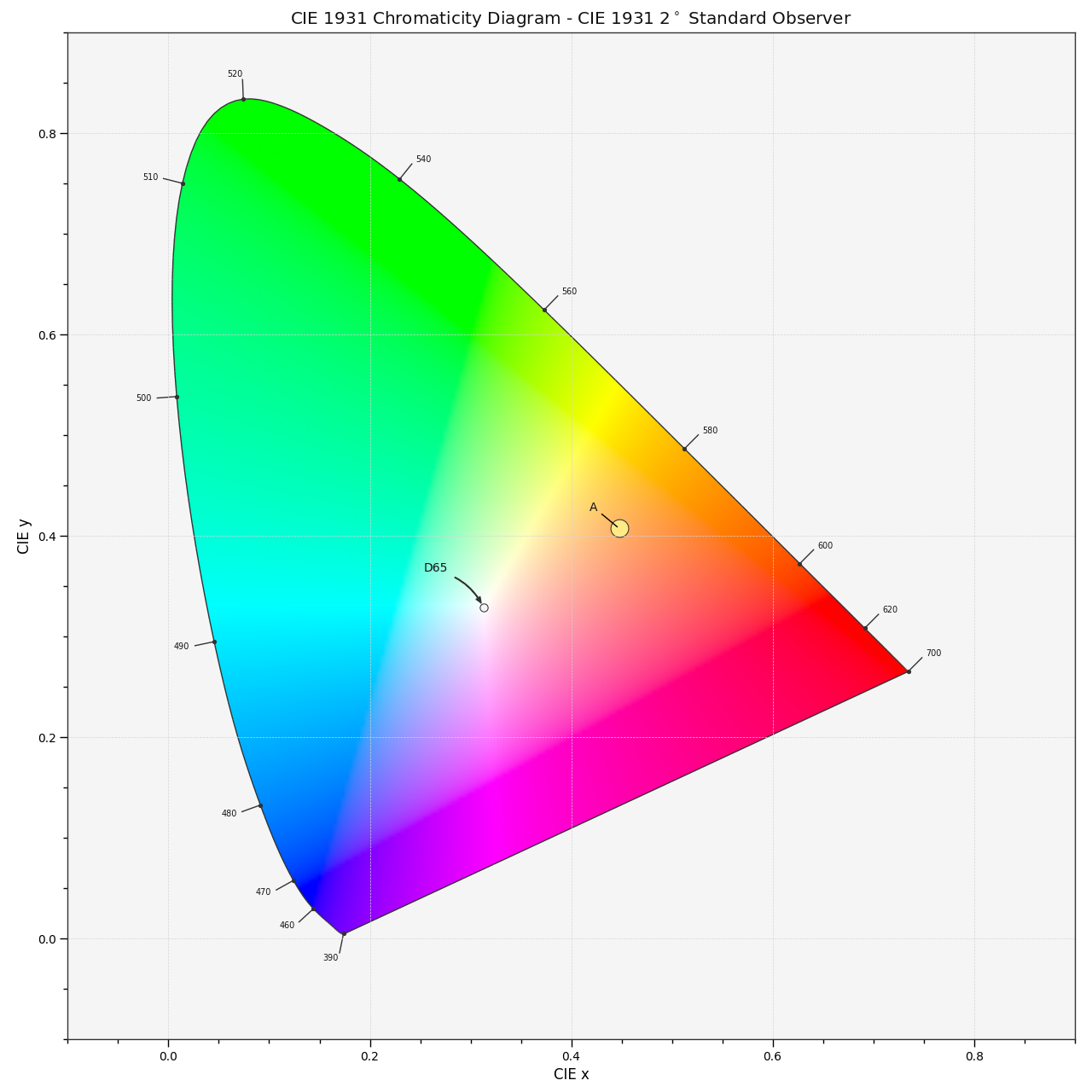# colour.plotting.diagrams.plot_sds_in_chromaticity_diagram#

colour.plotting.diagrams.plot_sds_in_chromaticity_diagram(sds: , cmfs: = 'CIE 1931 2 Degree Standard Observer', chromaticity_diagram_callable: Callable = plot_chromaticity_diagram, method: Literal['CIE 1931', 'CIE 1960 UCS', 'CIE 1976 UCS'] | str = 'CIE 1931', annotate_kwargs: dict | List[dict] | None = None, plot_kwargs: dict | List[dict] | None = None, **kwargs: Any) [source]#

Plot given spectral distribution chromaticity coordinates into the Chromaticity Diagram using given method.

Parameters:
Returns:

Current figure and axes.

Return type:

`tuple`

Examples

```>>> A = SDS_ILLUMINANTS["A"]
>>> D65 = SDS_ILLUMINANTS["D65"]
>>> annotate_kwargs = [
...     {"xytext": (-25, 15), "arrowprops": {"arrowstyle": "-"}},
...     {},
... ]
>>> plot_kwargs = [
...     {
...         "illuminant": SDS_ILLUMINANTS["E"],
...         "markersize": 15,
...         "normalise_sd_colours": True,
...         "use_sd_colours": True,
...     },
...     {"illuminant": SDS_ILLUMINANTS["E"]},
... ]
>>> plot_sds_in_chromaticity_diagram(
...     [A, D65], annotate_kwargs=annotate_kwargs, plot_kwargs=plot_kwargs
... )
...
(<Figure size ... with 1 Axes>, <...Axes...>)
```